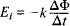# electromagnetic induction

(redirected from Magnetic mutual inductivity)
Also found in: Dictionary, Medical.

## electromagnetic induction:

see inductioninduction,
in electricity and magnetism, common name for three distinct phenomena. Electromagnetic induction is the production of an electromotive force (emf) in a conductor as a result of a changing magnetic field about the conductor and is the most important of the
.

## Electromagnetic induction

The production of an electromotive force either by motion of a conductor through a magnetic field in such a manner as to cut across the magnetic flux or by a change in the magnetic flux that threads a conductor. See Electromotive force (emf)

If the flux threading a coil is produced by a current in the coil, any change in that current will cause a change in flux, and thus there will be an induced emf while the current is changing. This process is called self-induction. The emf of self-induction is proportional to the rate of change of current.

The process by which an emf is induced in one circuit by a change of current in a neighboring circuit is called mutual induction. Flux produced by a current in a circuit A threads or links circuit B. When there is a change of current in circuit A, there is a change in the flux linking coil B, and an emf is induced in circuit B while the change is taking place. Transformers operate on the principle of mutual induction.

The phenomenon of electromagnetic induction has a great many important applications in modern technology.

## Electromagnetic Induction

the production of an electromotive force (induced emf) in a conducting circuit located in a variable magnetic field or moving in a constant magnetic field. The electric current induced by this emf is called an induced current. Electromagnetic induction was discovered by M. Faraday in 1831. According to Faraday’s law, the induced emf Ej in a circuit is directly proportional to the rate of change of the magnetic flux (the flux of the magnetic induction vector) ϕ through the surface S bounded by this circuit:Here ΔΦ is the change in the magnetic flux through the circuit over time Ac the proportionality coefficient k in the International System of Units is equal to k = 1 and in the cgs (Gauss) system, k = 1/c, where c is the velocity of light in a vacuum. The minus sign defines the direction of the induced current in accordance with Lenz’ rule: the direction of the induced current is such that the magnetic induction flux that it creates through the area bounded by the circuit tends to oppose the flux Φ variation that is responsible for the induced current.

In a constant magnetic field an induced emf arises only during the motion of the circuit in which the magnetic flux through the surface bounded by the circuit changes with time (that is, the circuit in its motion must intersect the lines of magnetic flux; when the motion is along the lines, the flux Φ will not change and no emf will arise). In this case the induced emf is equal to the work of the magnetic part of the Lorentz force needed to displace a unit charge along the closed circuit. If, however, a fixed conductor is in a constant magnetic field, the induced emf is equal to the work needed to displace a unit charge along the closed circuit; this work is performed by the forces of a circuital electric field, which, according to Maxwell’s equations, originates in space when a magnetic field changes with time. In a reference system, with respect to which the circuit is at rest, it is precisely this circuital electric field that induces the motion of charged particles, that is, the appearance of an induced current. Electromagnetic induction underlies the operation of electric current generators, in which mechanical energy is transformed into electrical; the operation of transformers and other equipment is also based on this phenomenon.

### REFERENCE

Kalashnikov, S. G. Elektrichestvo. Moscow, 1970. Chapter 9. (Obshchiikurs fiziki, vol. 2.)

G. IA. MIAKISHEV

## electromagnetic induction

[i¦lek·trō·mag′ned·ik in′dək·shən]
(electromagnetism)
The production of an electromotive force either by motion of a conductor through a magnetic field so as to cut across the magnetic flux or by a change in the magnetic flux that threads a conductor. Also known as induction.

## Electromagnetic induction

The production of an electromotive force either by motion of a conductor through a magnetic field in such a manner as to cut across the magnetic flux or by a change in the magnetic flux that threads a conductor. See Electromotive force (emf)

If the flux threading a coil is produced by a current in the coil, any change in that current will cause a change in flux, and thus there will be an induced emf while the current is changing. This process is called self-induction. The emf of self-induction is proportional to the rate of change of current.

The process by which an emf is induced in one circuit by a change of current in a neighboring circuit is called mutual induction. Flux produced by a current in a circuit A threads or links circuit B. When there is a change of current in circuit A, there is a change in the flux linking coil B, and an emf is induced in circuit B while the change is taking place. Transformers operate on the principle of mutual induction. See Transformer

The phenomenon of electromagnetic induction has a great many important applications in modern technology. See Generator, Induction heating, Motor, Servomechanism

Site: Follow: Share:
Open / Close